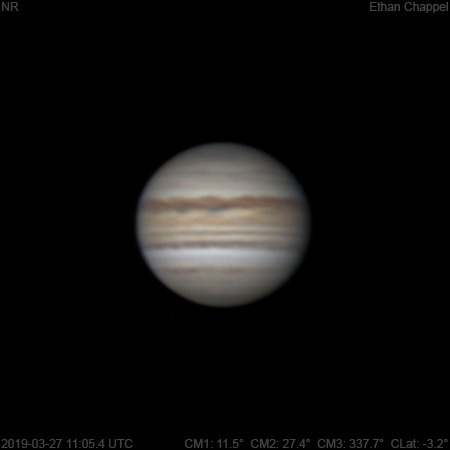# Jupiter 2019-03-27 11:05 UTC

CM1: 11.50°

CM2: 27.40°

CM3: 337.70°

CLat: -3.20°

Description

Seeing improved quite a bit since the last image. I was still manually tracking the planet with the hand controller.

Notes:

• The Equatorial Zone is still orange.
• The South Equatorial Belt is split by a white zone.

Equipment

ZWO ASI224MC

Celestron EdgeHD 8

Logs
```FireCapture v2.6  Settings
------------------------------------
Observer=Ethan Chappel
Camera=ZWO ASI224MC
Filter=RGB
Profile=Jupiter
Diameter=39.23"
Magnitude=-2.21
CMI=10.9° CMII=26.9° CMIII=337.2°  (during mid of capture)
FocalLength=2050mm (F/7)
Resolution=0.38"
Filename=2019-03-27-1105_4-EC-RGB-Jup.ser
Date=2019_03_27
Start=11_04_12.749
Mid=11_05_27.756
End=11_06_42.763
Start(UT)=11_04_12.749
Mid(UT)=11_05_27.756
End(UT)=11_06_42.763
Duration=150.014s
Date_format=yyyy_MM_dd
Time_format=HH_mm_ss
LT=UT -6h
Frames captured=9595
File type=SER
Binning=no
Bit depth=8bit
Debayer=yes
DebayerAlgorithm=NearestNeighbor
BayerPattern=RG
ROI=284x292
ROI(Offset)=0x0
FPS (avg.)=63
Shutter=15.00ms
Gain=210 (35%)
USBTraffic=100 (off)
WBlue=95 (off)
SoftwareGain=10 (off)
AutoGain=off
AutoHisto=75 (off)
Brightness=1 (off)
HardwareBin=off
FPS=100 (off)
HighSpeed=off
AutoExposure=off
WRed=55 (off)
Gamma=50 (off)
Histogramm(min)=0
Histogramm(max)=168
Histogramm=65%
Noise(avg.deviation)=0.08
AutoAlign=false
PreFilter=none
Limit=150 Seconds
Sensor temperature=20.1°C
```